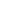# Add and subtract multiples of 10 and some ones within 100

EngageNY 60 min(s)
In this lesson, students continue to use relationships between the numbers to develop a variety of simplifying strategies to solve addition and subtraction within 100. They use multi-colored linking cubes to demonstrate how a difference between two numbers doesn't change if the same number of cubes is added to each subtrahend. Then students model this with tape diagrams. Finally, students use linking cubes and tape diagrams to demonstrate that adding a part of an addend to the other addend doesn't change the sum of the original two addends.